# 一、使用 print() 函数

## 1. 格式化输出字符串和整数

【示例1】输出字符串 AmoXiang，并计算、输出它的字符长度

 1 2 `str1 ``=` `"%s.length = %d"` `%` `(``"AmoXiang"``, ``len``(``"AmoXiang"``))` `print``(str1) ``# 输出AmoXiang.length = 8`

% 在字符串中表示格式化操作符，它后面必须附加一个格式化符号，具体说明如下表所示。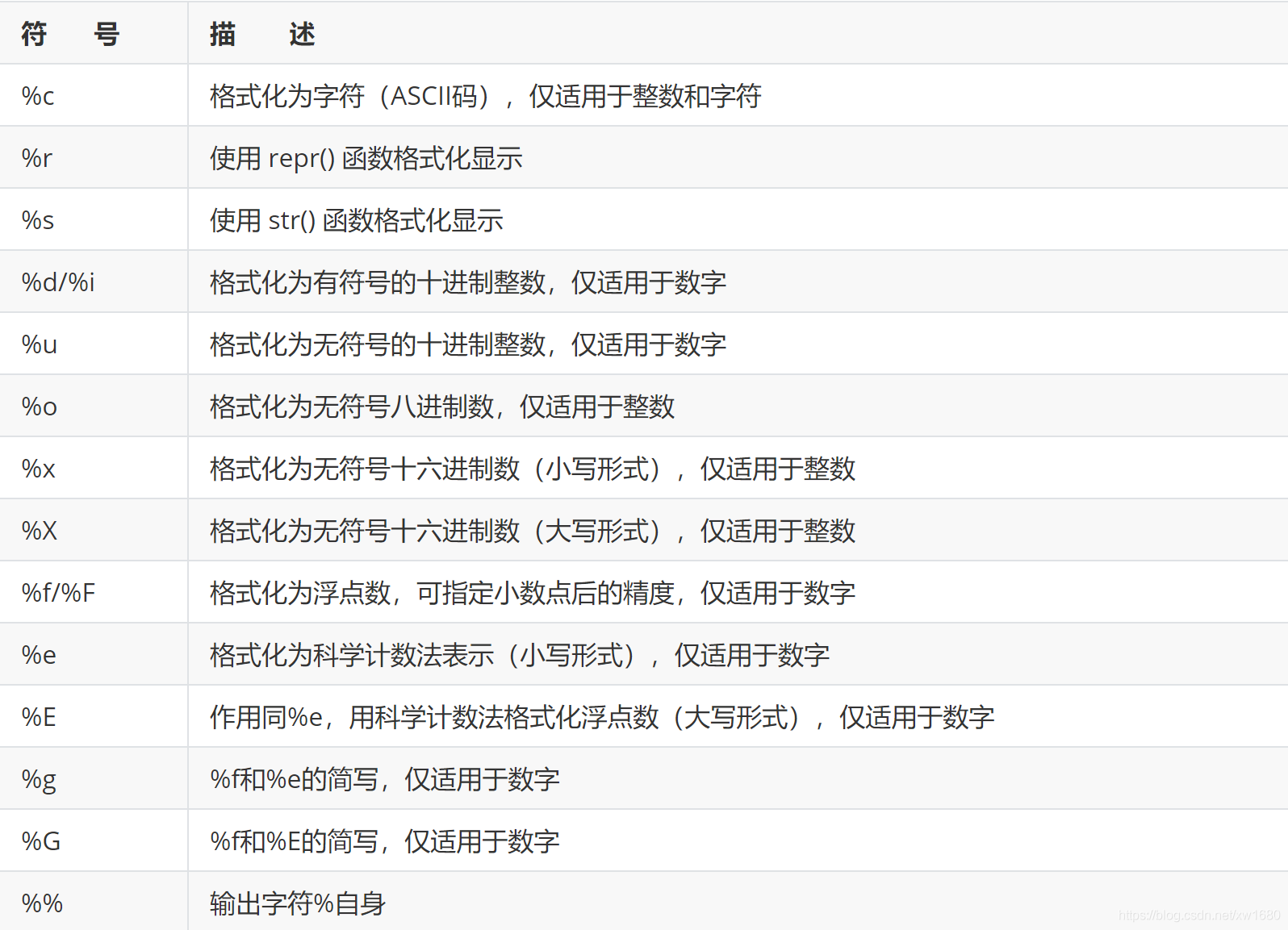%()元组可以包含一个或多个值，如变量或表达式，用来向字符串中%操作符传递值，元组包含元素数量、顺序都必须与字符串中%操作符一一对应，否则将抛出异常。%()元组必须位于字符串的后面，否则无效。如果字符串中只包含一个%操作符，那么也可以直接传递值。例如：

 1 2 `str1 ``=` `"AmoXiang.length = %d"` `%` `len``(``"AmoXiang"``)` `print``(str1) ``# 输出AmoXiang.length = 8`

## 2. 格式化输出不同进制数

【示例2】使用 print() 函数把数字输出为十六进制、十进制、八进制格式的字符串。

 1 2 3 `num ``=` `123` `# 输出：Hex = 7b Dec = 123 Oct=173` `print``(``"Hex = %x Dec = %d Oct=%o"` `%` `(num, num, num))`

``十进制转成其他进制# 10进制 -> 二进制# 11 - > 1011# 1011-> 8+2+1# print(bin(11)) # 0b1011 # 10进制 -> 八进制# print(oct(11)) # 0o13 # 10进制 -> 十六进制# print(hex(11)) # 0xb# print(hex(123)) # 0xb # 2.2.2 其他制转成其十进制# 二进制->10进制# print(int('0b1011',2)) # 11 # 二进制->8进制# print(int('0o13',8)) # 11 # 二进制->16进制# print(int('0xb',16)) # 11``

## 3. 格式化输出浮点数

【示例3】把数字输出为不同格式的浮点数字符串。

 1 2 3 4 5 6 `PI ``=` `3.141592653` `print``(``"pi1 = %10.3f"` `%` `PI) ``# 总宽度为10，小数位精度为3` `print``(``"pi2 = %.*f"` `%` `(``3``, PI)) ``# *表示从后面的元组中读取3，定义精度` `print``(``"pi3 = %010.3f"` `%` `PI) ``# 用0填充空白` `print``(``"pi4 = %-10.3f"` `%` `PI) ``# 左对齐，总宽度10个字符，小数位精度为3` `print``(``"pi5 = %+f"` `%` `PI) ``# 在浮点数前面显示正号`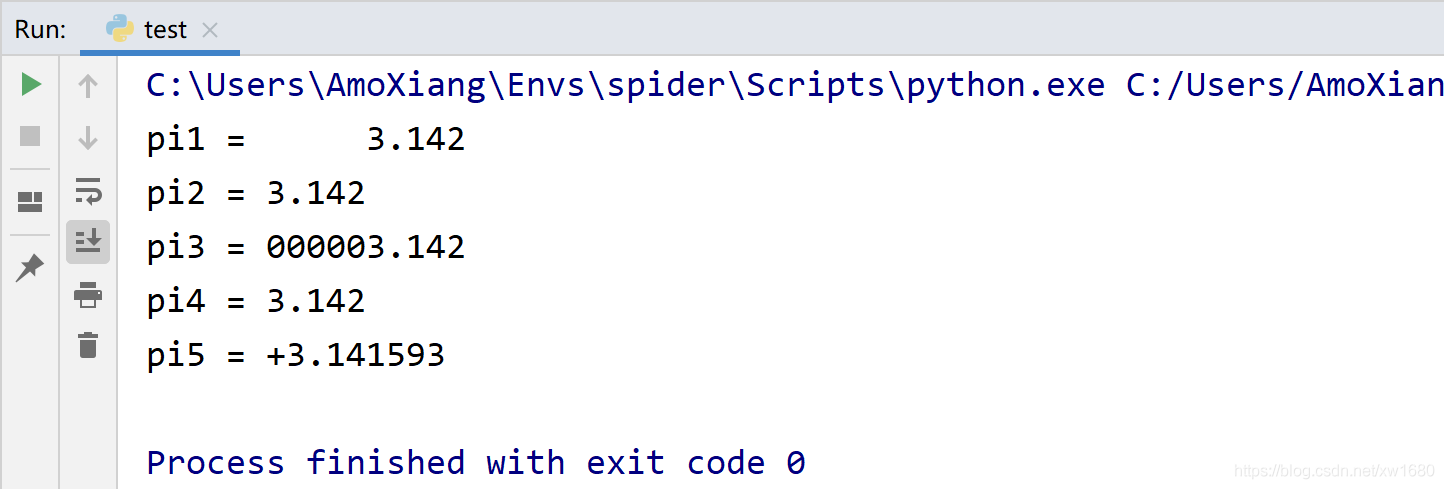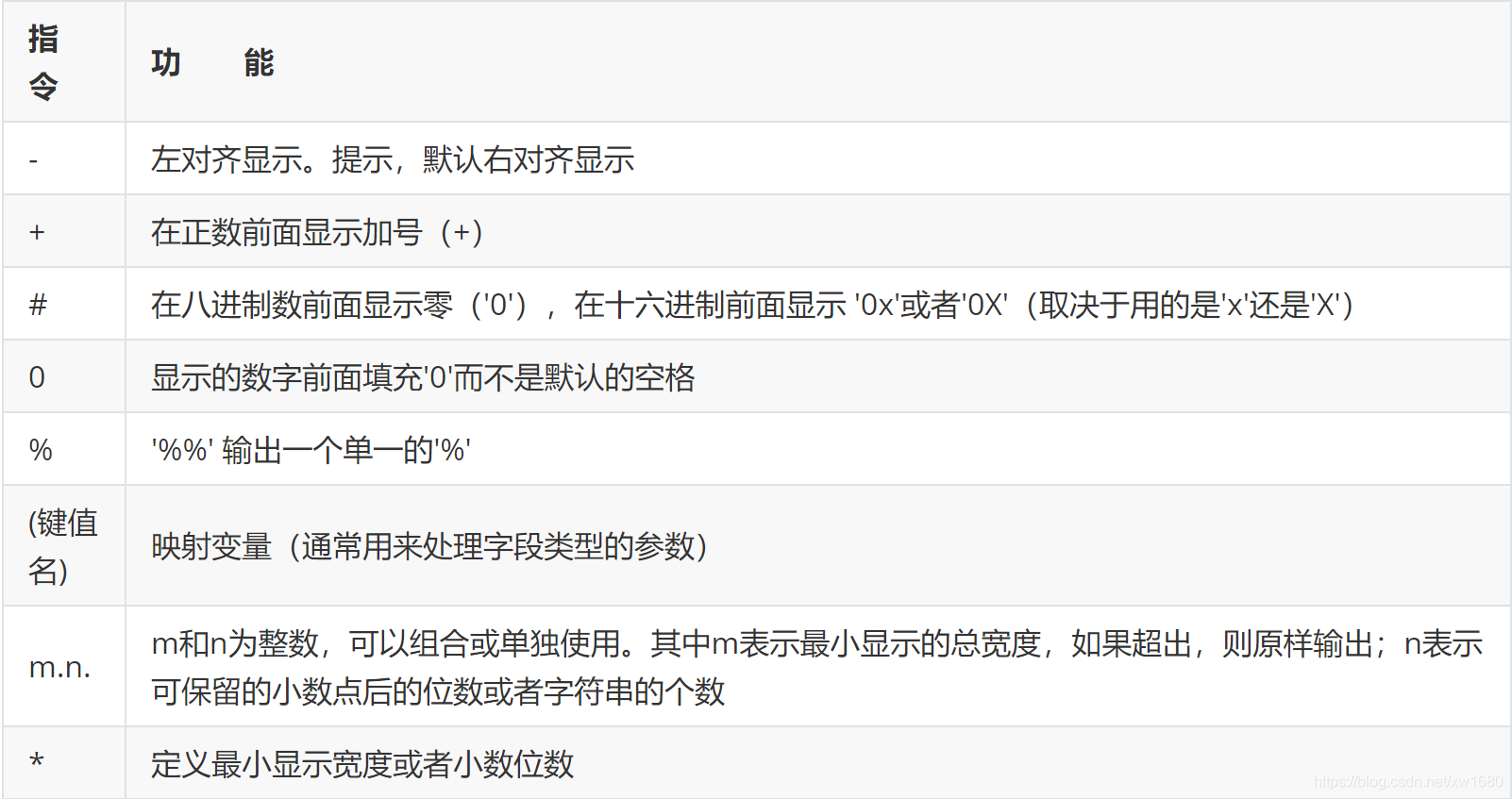# 二、使用 str.format() 方法

`%` 操作符是传统格式化输出的基本方法，从 Python 2.6 版本开始，为字符串数据新增了一种格式化方法 `str.format()`，它通过 `{}` 操作符和 `:` 辅助指令来代替 `%` 操作符。
【示例1】通过位置索引值

 1 2 3 `print``(``'{0} {1}'``.``format``(``'Python'``, ``3.7``)) ``# Python 3.7` `print``(``'{} {}'``.``format``(``'Python'``, ``3.7``)) ``# Python 3.7` `print``(``'{1} {0} {1}'``.``format``(``'Python'``, ``3.7``)) ``# 3.7 Python 3.7`

【示例2】通过关键字索引值

 1 2 `# 输出：Amo年龄是18岁。` `print``(``'{name}年龄是{age}岁。'``.``format``(age``=``18``, name``=``"Amo"``))`

【示例3】通过下标进行索引

 1 2 3 `L ``=` `[``"Jason"``, ``30``]` `# 输出：Jason年龄是30岁。` `print``(``'{0}年龄是{0}岁。'``.``format``(L))`

## 1. 填充与对齐

`:` 符号后面可以附带填充的字符，默认为空格， `^、<、>` 分别表示居中、左对齐、右对齐，后面附带宽度限定值。

【示例4】下面示例设计输出 8 位字符，并分别设置不同的填充字符和值对齐方式。

 1 2 3 `print``(``'{:>8}'``.``format``(``'1'``)) ``# 总宽度为8，右对齐，默认空格填充` `print``(``'{:0>8}'``.``format``(``'1'``)) ``# 总宽度为8，右对齐，使用0填充` `print``(``'{:a<8}'``.``format``(``'1'``)) ``# 总宽度为8，左对齐，使用a填充`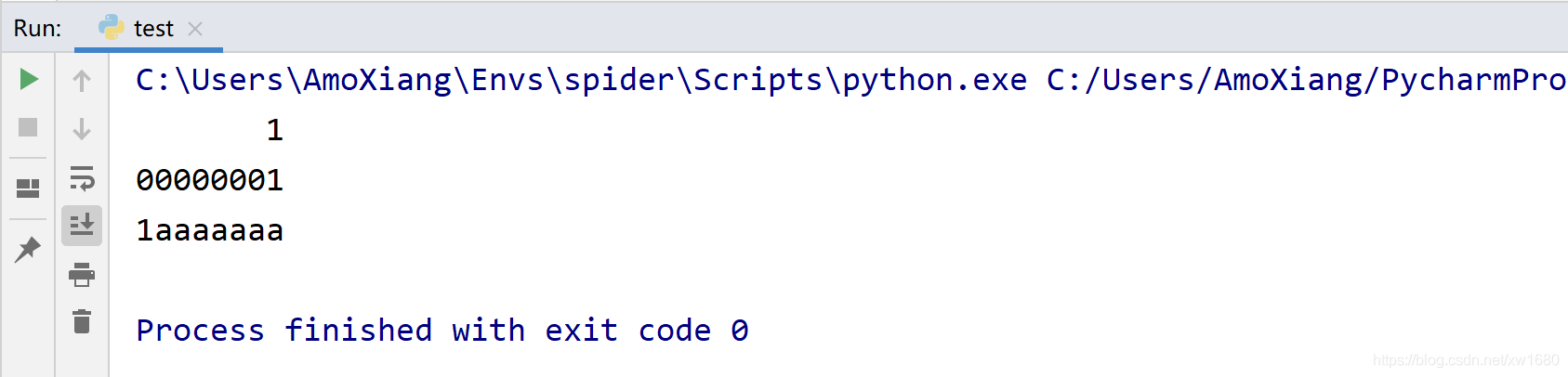## 2. 精度与类型f

【示例5】f 与 float 类型数据配合使用

 1 `print``(``'{:.2f}'``.``format``(``3.141592653``)) ``# 输出结果：3.14`

## 3. 进制数字输出

【示例6】使用b、d、o、x 分别输出二进制、十进制、八进制、十六进制数字。

 1 2 3 4 5 `num ``=` `100` `print``(``'{:b}'``.``format``(num)) ``# 1100100` `print``(``'{:d}'``.``format``(num)) ``# 100` `print``(``'{:o}'``.``format``(num)) ``# 144` `print``(``'{:x}'``.``format``(num)) ``# 64`

## 4. 千位分隔输出

【示例7】使用逗号（,）输出金额的千分位分隔符。

 1 `print``(``'{:,}'``.``format``(``1234567890``)) ``# 1,234,567,890`

# 三、详解 format() 函数

format()函数可以对数据进行格式化处理操作。语法格式如下：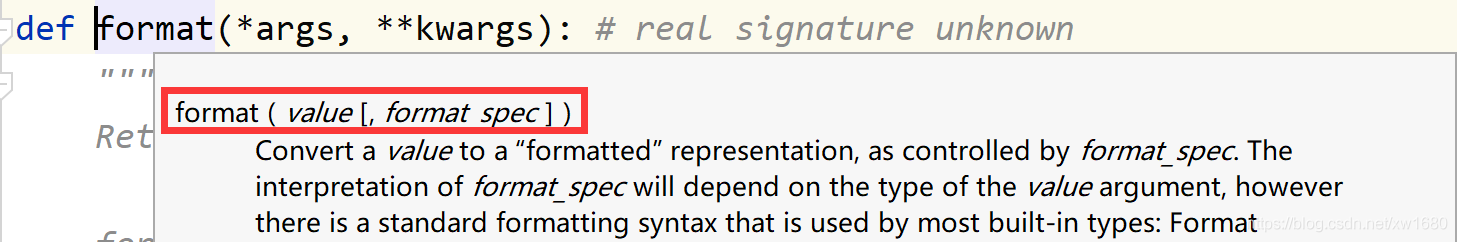format_spec为格式化解释。当参数 format_spec 为空时，等同于函数 str(value) 的方式。value 为要转换的数据。format_ spec 可以设置非常复杂的格式转换参数，生成比较完备的数据格式处理模板。format_spec 的编写方式如下形式：

 1 `format_spec ::``=` `[[fill]align][sign][``#][width][,][.precision][type]`

format_spec 格式控制符可以分为文字对齐、填充值、标志设置、格式化、类型转换、千位符（数字分组）等主要应用。细化分类说明如下图所示：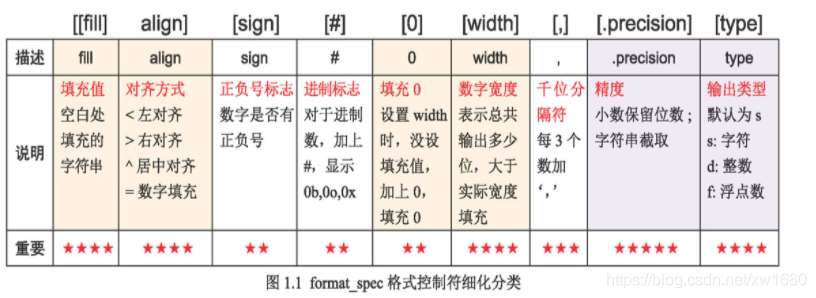(1) fill(填充值)：此处可以填写任何字符，通常与align、width一起使用，实现指定位数字符填充，通常格式如图1.2所示，填充效果及位置如图1.3所示。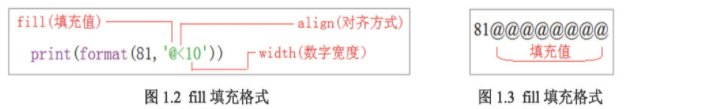1 2 3 4 `# 81@@@@@@@@` `print``(``format``(``81``, ``'@<10'``)) ``# 用@符号填充，宽度为10个空格` `# @@@@@@@@81` `print``(``format``(``81``, ``'@>10'``)) ``# 用@符号填充，宽度为10个空格`

(2) align(对齐方式)：指在 width(数字宽度) 内输出时的对齐方式，分别使用 `<、>、^、=` 4个符号表示左对齐、右对齐、居中对齐和数字填充（只用于数字，在符号后进行补齐)。
(3) width(数字宽度)：指设定输出字符宽度，如果数据的实际位数比 width 指定宽度值大，则使用数据实际的长度。如果该值的实际位数小于指定宽度，则位数将设定的 fill 值填充或设定的 0 值填充，如果没有设置填充值，则用空格填充。

 1 2 3 4 5 6 7 8 9 10 11 12 13 14 15 `s ``=` `"PYTHON"` `print``(``format``(s, ``'10'``)) ``# 没有标志符，如果是字符串则默认左对齐，不足宽度部分默认用空格填充` `print``(``format``(``13.14``, ``'10'``)) ``# 没有标志符，如果是数字则默认右对齐，不足宽度部分默认用空格填充` `print``(``format``(s, ``'0>10'``)) ``# 右对齐，不足指定宽度部分用0填充` `print``(``format``(s, ``'>04'``)) ``# 右对齐，因字符实际宽度大于指定宽度4，不用填充` `print``(``format``(s, ``'*>10'``)) ``# 右对齐，不足部分用"*"填充` `print``(``format``(s, ``'>010'``)) ``# 右对齐，不足部分用0填充` `print``(``format``(s, ``'>10'``)) ``# 右对齐，默认用空格填充` `print``(``format``(s, ``'<10'``)) ``# 左对齐，默认用空格填充` `print``(``format``(s, ``'<010'``)) ``# 左对齐，不足部分用0填充` `print``(``format``(s, ``'@^10'``)) ``# 中间对齐，不足部分用'@'填充，宽度为10个空格` `print``(``format``(``13.14``, ``'0<10'``)) ``# 左对齐，不足部分用0填充` `print``(``format``(``13.14``, ``'@^10'``)) ``# 中间对齐，不足部分用@填充` `print``(``format``(``13.14``, ``'0>10'``)) ``# 右对齐，不足部分用0填充` `print``(``format``(``-``13.14``, ``'0=10'``)) ``# 右对齐，符号后面不足部分用0填充`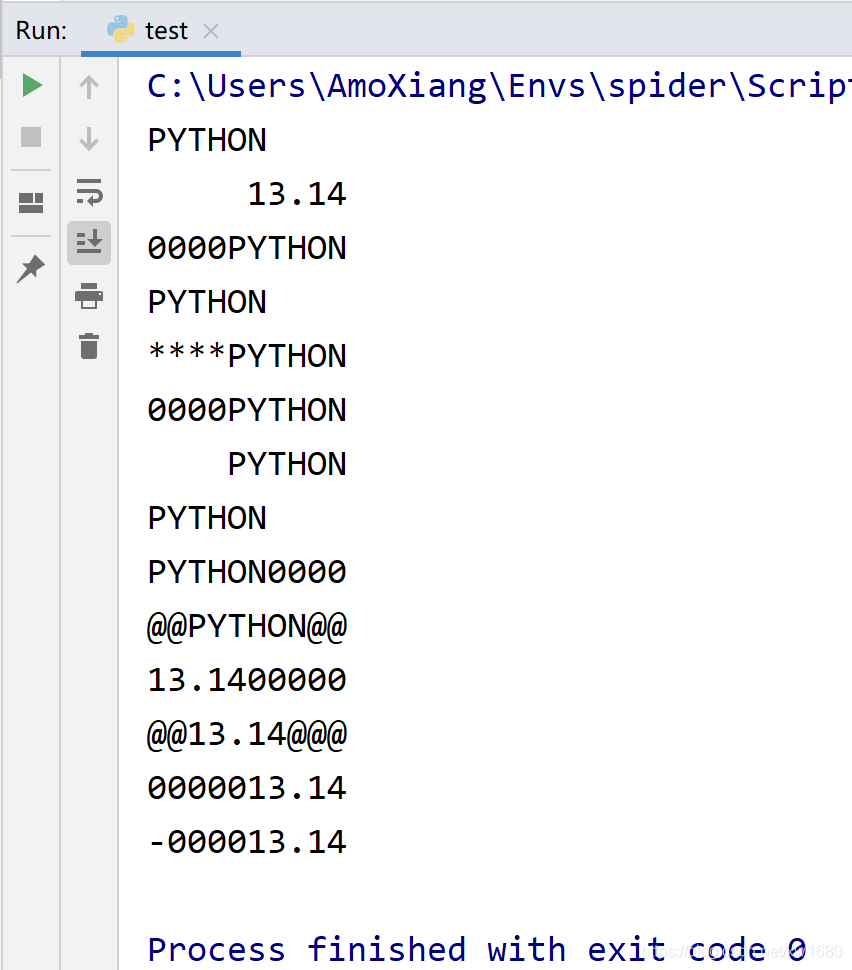(4) precision(精度)：精度由小数点 `.` 开头。对于浮点数，精度表示小数部分输出的有效位数。对于字符串，精度表示输出的最大长度。precision(精度) 通常和 type(类型) 配合起来使用。
(5) type(类型)：表示输出字符串、整数和浮点数类型的格式规则，默认为字符型 s。对于整数类型，输出格式包括 7 种：

b：输出整数的二进制方式。c：输出整数对应的 Unicode 字符。d：输出整数的十进制方式。n：输出整数的十进制方式。o：输出整数的八进制方式。x：输出整数的小写十六进制方式。当值为整数时和 d 类似，值为浮点数时和 g 类似。不同之处在于 n 会使用当前区域设置来插入适当数字分隔字符。X：输出整数的大写十六进制方式。

e：输出浮点数对应的小写字母 e 的指数形式。E：输出浮点数对应的大写字母 E 的指数形式。f：输出浮点数的浮点表示形式，默认保留 6 位小数。F：输出浮点数的浮点表示形式，默认保留 6 位小数，无穷大转换成大写字母 INF。g：自动调整将整数、浮点数转换成浮点型或科学记数法表示(超过 6 位数用科学记数法)，并将其格式化到指定位置（如果是科学计数则是 e)。G：自动调整将整数、浮点数转换成浮点型或科学记数法表示(超过 6 位数用科学记数法)，并将其格式化到指定位置（如果是科学计数则是 E)。%：输出浮点数的百分形式。 运用一：格式转换

 1 2 3 4 5 6 7 8 `import` `datetime` `# 使用format()函数将浮点数转换成字符，结果为：'3.14'` `print``(``format``(``3.14``))` `# 使用str()函数将浮点数转换成字符，结果为：'3.14'` `print``(``str``(``3.14``))` `# 将日期格式化为字符，结果为：'2021-01-17 05:25:02'` `print``(``format``(datetime.datetime.utcnow().strftime(``"%Y-%m-%d %H:%M:%S"``)))`

 1 2 3 4 5 6 7 8 9 10 11 `print``(``format``(``12.2``, ``'f'``)) ``# 转换成浮点数，默认为小数保留6位，输出：12.200000` `print``(``format``(``12``, ``'d'``)) ``# 转换成十进制，输出：12` `print``(``format``(``13``)) ``# 不带参数默认为十进制，输出：13` `print``(``format``(``13``, ``'n'``)) ``# 转换成十进制数，输出：13` `print``(``format``(``13``, ``'b'``)) ``# 转换成二进制，输出：1101` `print``(``format``(``65``, ``'c'``)) ``# 转换Unicode成字符，输出：A` `print``(``format``(``97``, ``'c'``)) ``# 转换Unicode成字符，输出：a` `print``(``format``(``8750``, ``'c'``)) ``# 转换Unicode成字符，输出：∮` `print``(``format``(``12``, ``'o'``)) ``# 转换成八进制，输出：14` `print``(``format``(``12``, ``'x'``)) ``# 转换成十六进制小写字母表示，输出：c` `print``(``format``(``12``, ``'X'``)) ``# 转换成十六进制大写字母表示，输出：C`

 1 2 3 `print``(``format``(``1``, ``'0>3'``)) ``# 输出：001` `print``(``format``(``1``, ``'>03'``)) ``# 输出：001` `print``(``format``(``15``, ``'0>5'``)) ``# 输出：00015`

 1 2 3 4 5 6 7 8 9 10 `import` `datetime` `# 时间+编号` `wx ``=` `datetime.datetime.now().date()` `now ``=` `datetime.datetime.now()` `print``(``str``(wx), ``format``(``1``, ``'0>3'``)) ``# 年-月-日 +3位编号，输出：2021-01-17 001` `print``(``format``(now, ``'%Y-%m-%d'``), ``format``(``1``, ``'0>3'``)) ``# 年-月-日 +3位编号，输出：2021-01-17 001` `print``(``format``(now, ``'%Y%m%d'``), ``'NO'` `+` `format``(``1``, ``'0>3'``)) ``# 年-月-日+NO+3位编号，输出：20210117 NO001` `print``(``format``(now, ``'%d'``), ``'NO'` `+` `format``(``1``, ``'0>3'``)) ``# 日期 +NO+3位编号，输出：17 NO001` `print``(``format``(now, ``'%H%M'``), ``'NO'` `+` `format``(``1``, ``'0>3'``)) ``# 时-+分 +NO+3位编号，输出：1411 NO001`

 1 2 3 `# 批量生成编号` `for` `i ``in` `range``(``1``, ``6``):` ` ``print``(``format``(i, ``'0>2'``))`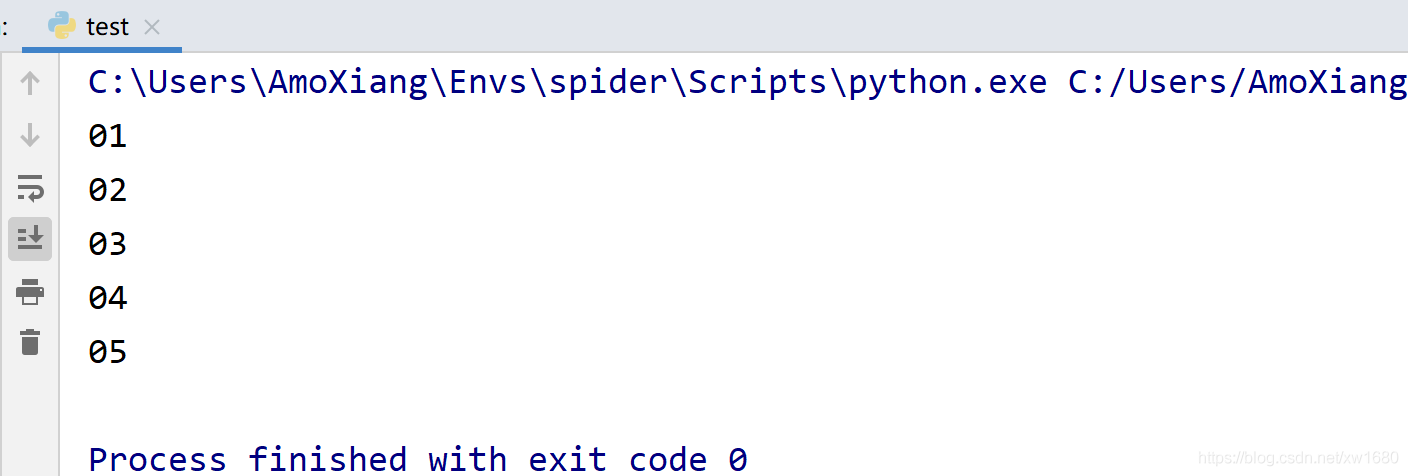1 2 3 4 5 `# 格式化列表编号` `# 对已有非编号数字进行格式化编号` `nba ``=` `{``1``: ``'伦纳德'``, ``2``: ``'哈登'``, ``3``: ``'乔治'``}` `for` `key, value ``in` `nba.items():` ` ``print``(``format``(key, ``'0>3'``), value)`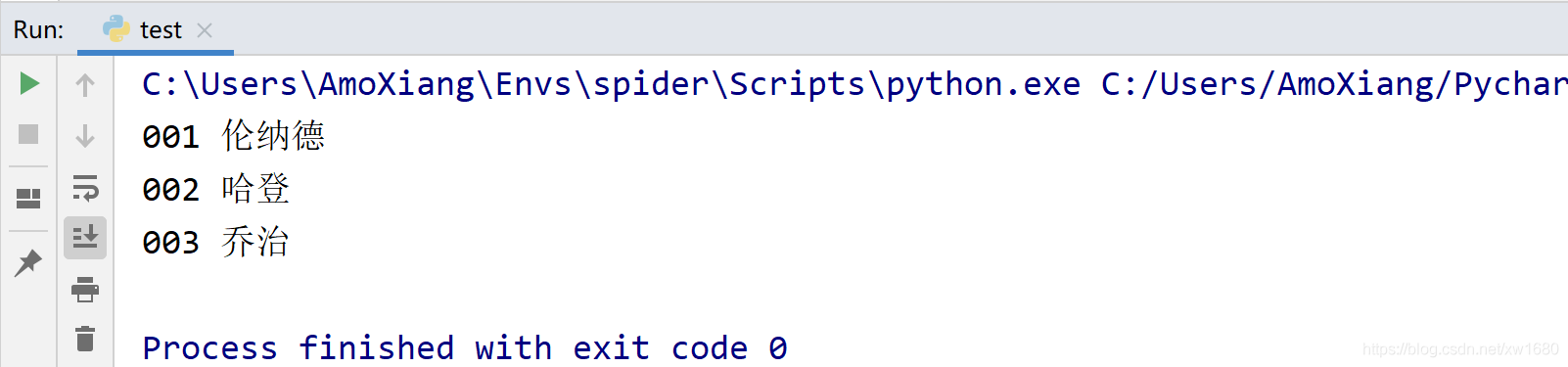1 2 3 4 5 `for` `i ``in` `range``(``65``, ``69``):` ` ``for` `j ``in` `range``(``1``, ``6``):` ` ``data ``=` `chr``(i) ``+` `format``(j, ``'0>3'``) ``+` `' '` ` ``print``(data, end``=``'')` ` ``print``()`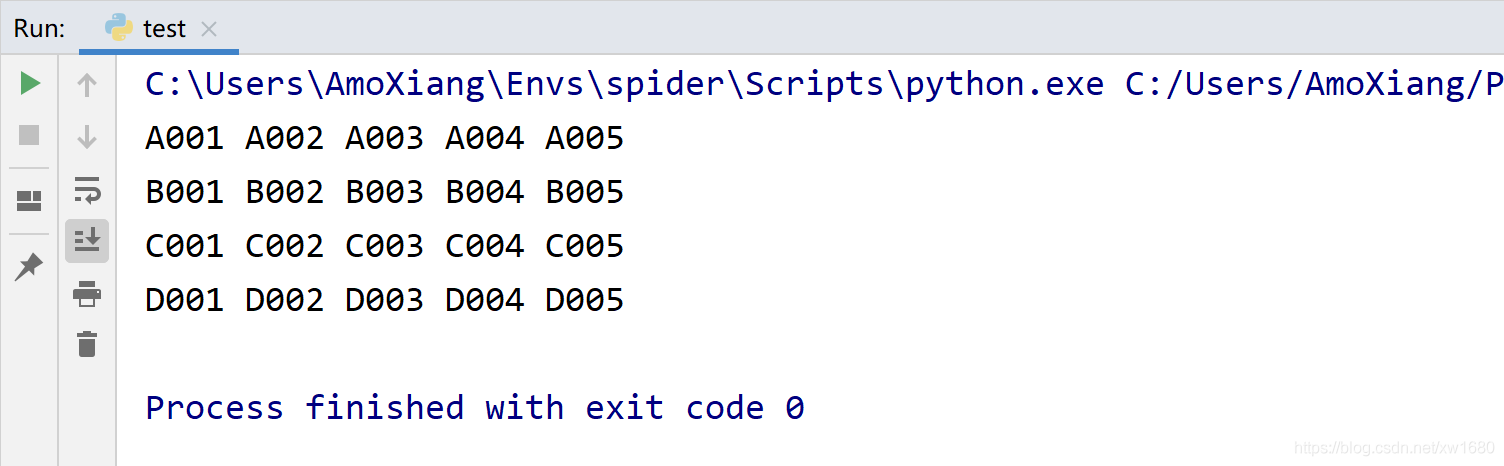1 2 3 4 5 6 7 8 9 10 11 12 13 14 15 16 17 18 19 20 21 22 23 24 25 `print``(``format``(``81``, ``'8d'``)) ``# 8位整数显示，不足部分整数前用空格填充` `print``(``format``(``81``, ``'+d'``)) ``# 格式化为带符号整数显示数据` `print``(``format``(``-``81``, ``'8d'``)) ``# 格式化为8位带符号整数显示，补位空格放到符号前` `print``(``format``(``81``, ``'=8d'``)) ``# 格式化为8位正整数，用空格补位` `print``(``format``(``-``81``, ``'=8d'``)) ``# 格式化为8位负整数，不足部分在负号后填充` `print``(``format``(``81``, ``'+8d'``)) ``# 格式化为8位正整数，不足部分在符号前填充` `print``(``format``(``-``81``, ``'8d'``)) ``# 格式化为8位负整数，不足部分在符号前填充` `print``(``format``(``81``, ``'>10'``)) ``# 右对齐，宽度为10个字符` `print``(``format``(``81``, ``'<10'``)) ``# 左对齐，宽度为10个字符` `print``(``format``(``81``, ``'010'``)) ``# 用0填充空格，宽度为10个字符` `print``(``format``(``81``, ``'@<10'``)) ``# 用“@”填充空格，宽度为10个字符` `print``(``format``(``81``, ``'@>10'``)) ``# 用“@”填充空格，宽度为10个字符` `print``(``format``(``+``81``, ``'=10'``)) ``# 右对齐，宽度为10个字符` `print``(``format``(``81``, ``'0^10'``)) ``# 用0填充空格，宽度为10个字符` `s ``=` `125` `print``(``format``(s, ``'0>10'``)) ``# 右对齐，不足指定宽度部分用0填充` `print``(``format``(s, ``'>04'``)) ``# 右对齐，不足指定宽度部分用0填充` `print``(``format``(s, ``'*>10'``)) ``# 右对齐，不足指定宽度部分用“*”填充` `print``(``format``(s, ``'>010'``)) ``# 右对齐，指定0标志位填充` `print``(``format``(s, ``'>10'``)) ``# 右对齐，没指定填充值，用默认值空格填充` `print``(``format``(s, ``'+^30'``)) ``# 居中对齐，用“+”填充不足部分` `print``(``format``(s, ``'*<8'``)) ``# 右对齐，不足指定宽度部分用“*”填充` `print``(``format``(s, ``'08'``)) ``# 右对齐，指定0标志位填充`

 1 2 3 4 5 6 7 8 9 10 11 12 13 14 15 16 17 18 `print``(``format``(``628``, ``'.1f'``)) ``# 格式化为保留1位小数的浮点数，输出：628.0` `print``(``format``(``628``, ``'.2f'``)) ``# 格式化为保留2位小数的浮点数，输出：628.00` `print``(``format``(``3.14159``, ``'.1f'``)) ``# 格式化为保留1位小数的浮点数，输出：3.1` `print``(``format``(``3.14159``, ``'.2f'``)) ``# 格式化为保留2位小数的浮点数，输出：3.14` `print``(``format``(``3.14159``, ``'.5f'``)) ``# 格式化为保留5位小数的浮点数，输出：3.14159` `print``(``format``(``-``3.14159``, ``'.3f'``)) ``# 格式化为保留3位小数的浮点数，输出：-3.142` `print``(``format``(``3.1415926535898``, ``'f'``)) ``# 默认精度保留6位小数，输出：3.141593` `# 默认精度保留6位小数，不足部分用空格填充，输出：3.141590` `print``(``format``(``3.14159``, ``'f'``))` `print``(``format``(``3.14159``, ``'+.3f'``)) ``# 格式化为保留3位小数带符号的浮点数` `print``(``format``(``3.14159``, ``'>8.2f'``)) ``# 右对齐，保留2位小数` `print``(``format``(``3.14159``, ``'<10.2f'``)) ``# 左对齐，宽度为10，保留2位小数，不足部分用空格填充` `print``(``format``(``3.14159``, ``'<.3f'``)) ``# 左对齐，保留3位小数` `print``(``format``(``3.14159``, ``'@>10.3f'``)) ``# 右对齐，用“@”填充不足位置` `print``(``format``(``-``3.14159``, ``'=10.2f'``)) ``# 格式化为保留2位小数的10位数，默认用空格填充` `print``(``format``(``-``3.14159``, ``'0=10.2f'``)) ``# 格式化为保留2位小数的10位数，空格用0填充` `print``(``format``(``3.14159``, ``'0^10.2f'``)) ``# 保留2位小数的10位数，居中显示，空格用0填充`

 1 2 3 4 5 6 7 8 `print``(``format``(``0.161896``, ``'%'``)) ``# 将小数格式化成百分数，输出：16.189600%` `print``(``format``(``0.161896``, ``'.2%'``)) ``# 格式化为保留2位小数的百分数，输出：16.19%` `print``(``format``(``0.0238912``, ``'.6%'``)) ``# 格式化为保留6位小数的百分数，输出：2.389120%` `print``(``format``(``2` `/` `16``, ``'.2%'``)) ``# 格式化为保留2位小数的百分数，输出：12.50%` `print``(``format``(``3.1415926``, ``'.1%'``)) ``# 格式化为保留1位小数的百分数，输出：314.2%` `print``(``format``(``0.161896``, ``'.0%'``)) ``# 格式化为保留整数的百分数，输出：16%` `print``(``format``(``0.0238912``, ``'8.6%'``)) ``# 格式化为保留6位小数的八位百分数，输出：2.389120%` `print``(``format``(``0.0238912``, ``'>8.3%'``)) ``# 格式化为保留3位小数的八位百分数，输出：2.389%`

 1 2 3 4 5 6 7 8 9 10 11 12 13 `#####e和E` `print``(``format``(``3141592653589``, ``'e'``)) ``# 科学记数法，默认保留6位小数，输出：3.141593e+12` `print``(``format``(``3.14``, ``'e'``)) ``# 科学记数法，默认保留6位小数，输出：3.140000e+00` `print``(``format``(``3.14``, ``'0.4e'``)) ``# 科学记数法，默认保留4位小数，输出：3.1400e+00` `print``(``format``(``3141592653589``, ``'0.2e'``)) ``# 科学记数法，保留2位小数，输出：3.14e+12` `print``(``format``(``3141592653589``, ``'0.2E'``)) ``# 科学记数法，保留2位小数，采用大写E表示，输出：3.14E+12` `#####g和G` `print``(``format``(``3.14e``+``1000000``, ``'F'``)) ``# 无穷大转换成大写字母，输出：INF` `print``(``format``(``3141592653589``, ``'g'``)) ``# 科学记数法，保留2位小数，输出：3.14159e+12` `print``(``format``(``314``, ``'g'``)) ``# 科学记数法，保留2位小数，输出：314` `print``(``format``(``3141592653589``, ``'0.2g'``)) ``# 科学记数法，保留2位有效数字，采用小写e表示，输出：3.1e+12` `print``(``format``(``3141592653589``, ``'G'``)) ``# 科学记数法，保留5位小数，采用大写E表示，输出：3.14159E+12` `print``(``format``(``3.14e``+``1000000``, ``'g'``)) ``# 小数点计数法，无穷大转换成小写字母，输出：inf`

format() 函数还能用来作金额的千位分隔符。如果要实现金额前面带上相关货币的符号形式的功能，需要在该函数前面手动加上相应货币符号。如：

 1 2 3 4 5 6 `print``(``'\$'` `+` `format``(``1201398.2315``, ``'.2f'``)) ``# 添加美元符号，小数保留2位` `print``(``chr``(``36``) ``+` `format``(``1201398.2315``, ``'.2f'``)) ``# ASCII码添加美元符号，小数保留2位` `print``(``'¥'` `+` `format``(``78088888``, ``','``)) ``# 添加人民币符号，用千位分隔符区分金额` `print``(``'£'` `+` `format``(``7908.2315``, ``'.2f'``)) ``# 添加英镑符号，用千位分隔符进行区分` `print``(``'€'` `+` `format``(``7908.2315``, ``',.2f'``)) ``# 添加欧元符号，保留两位小数，千位分隔` `print``(``chr``(``0x20ac``) ``+` `format``(``1201398.2315``, ``',f'``)) ``# 使用十六进制编码添加欧元符号`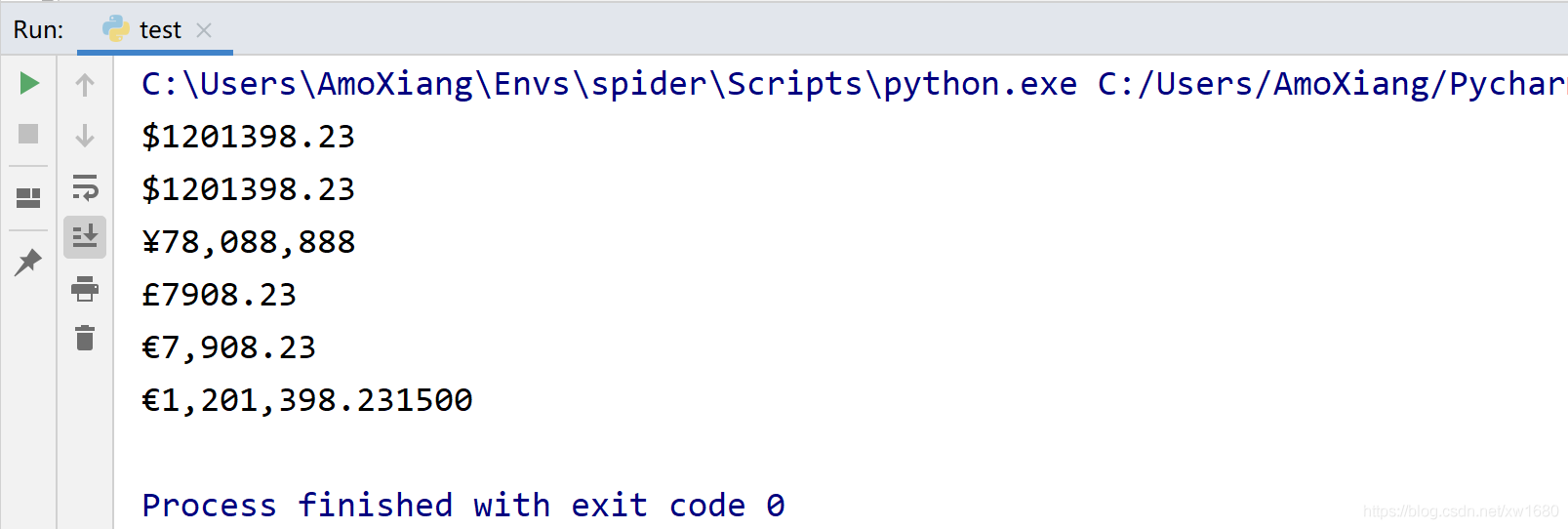1 2 3 4 5 6 7 8 9 10 11 `print``(``format``(``'PYTHON'``, ``'M^20.3'``)) ``# 截取3个字符，宽度为20居中，不足用M填充` `print``(``format``(``"PYTHON"``, ``'10'``)) ``# 默认居左显示，不足部分用空格填充` `print``(``format``(``'blog.csdn.net'``, ``'.3'``)) ``# 截取3个字符，默认居左显示` `print``(``format``(``"PYTHON"``, ``'>10'``)) ``# 居右显示，不足部分用空格填充` `s ``=` `'blog.csdn.net'` `print``(``format``(s, ``'0>20'``)) ``# 右对齐，不足指定宽度部分用0填充` `print``(``format``(s, ``'>4'``)) ``# 右对齐，因字符实际宽度大于指定宽度4，不用填充` `print``(``format``(s, ``'*>20'``)) ``# 右对齐，不足指定宽度部分用*填充` `print``(``format``(s, ``'>020'``)) ``# 右对齐，指定0标志位填充` `print``(``format``(s, ``'>20'``)) ``# 右对齐，没指定填充值，用默认值空格填充` `print``(``format``(s, ``'+^30'``)) ``# 居中对齐，用+填充不足部分`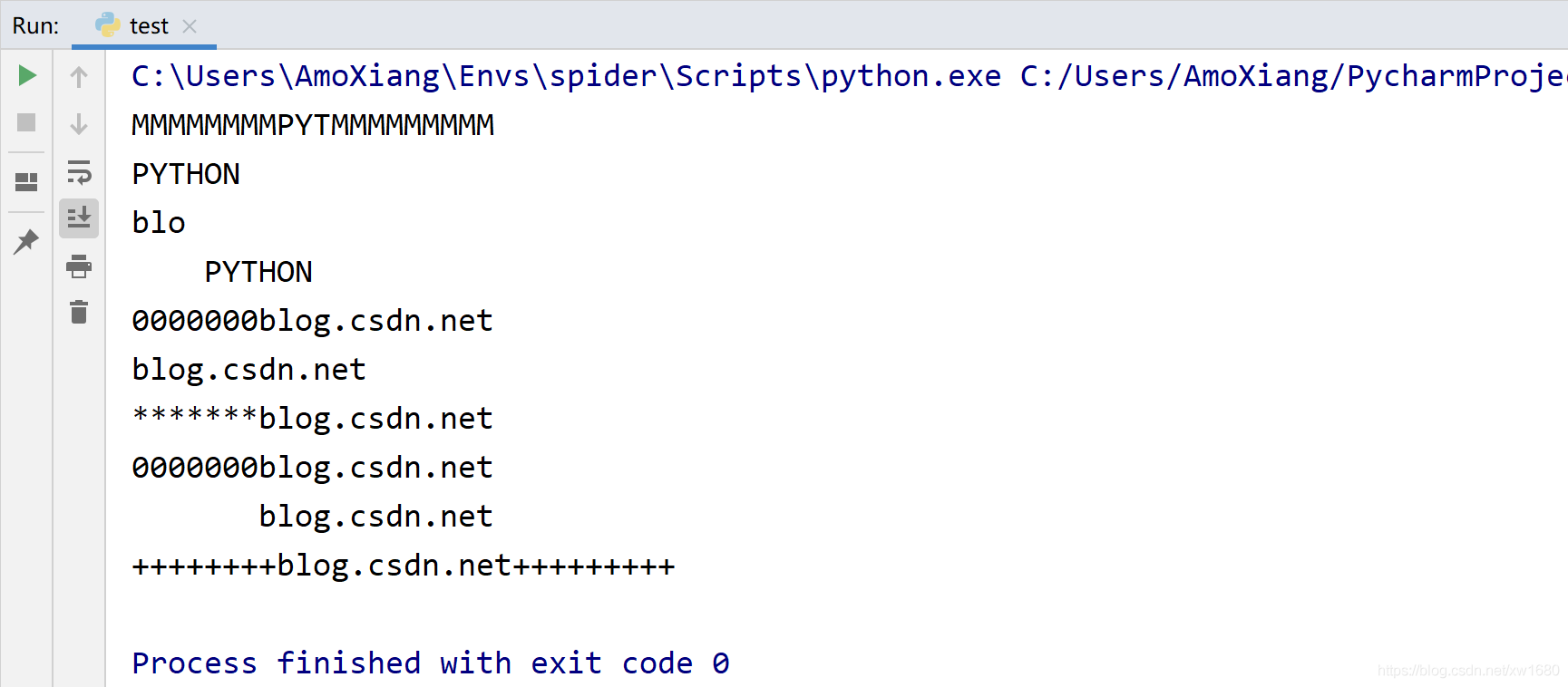b：二进制。将数字以 2 为基数进行输出。d：十进制整数。将数字以 10 为基数进行输出。o：八进制。将数字以 8 为基数进行输出。x：十六进制。将数字 以16 为基数进行输出，9 以上的数字用小写字母。

 1 2 3 4 5 6 7 8 9 10 11 12 13 14 15 16 17 18 19 20 21 `print``(``format``(``77``)) ``# 格式参数为空，默认为十进制` `print``(``format``(``77``, ``'d'``)) ``# 原来是十进制数，转换后为原值` `print``(``format``(``-``77``, ``'d'``)) ``# 原来是十进制数，转换后为原值` `print``(``format``(``77``, ``'8d'``)) ``# 转换为8位十进制数，空余部分用空格填充` `print``(``format``(``-``77``, ``'8d'``)) ``# 转换为8位十进制数，负数在负号前填充空余部分空格` `print``(``format``(``77``, ``'+8d'``)) ``# 转换为8位带符号十进制数，在符号前填充空余部分空格` `print``(``format``(``-``77``, ``'08d'``)) ``# 转换为8位十进制数，负数在负号前填充空余部分空格` `print``(``format``(``77``, ``'+08d'``)) ``# 转换为8位带符号十进制数，在符号前填充空余部分空格` `print``(``format``(``-``77``, ``'#8d'``)) # 转换为``8``位十进制数，加进制标志` `print``(``format``(``-``77``, ``'=8d'``)) ``# 转换为8位十进制数，空余部分填充空格` `print``(``format``(``+``77``, ``'=8d'``)) ``# 转换为8位十进制数，空余部分填充空格` `print``(``format``(``+``77``, ``'*=8d'``)) ``# 转换为8位十进制数，空余部分填充*` `print``(``format``(``+``77``, ``'*=+8d'``)) ``# 转换为8位带符号十进制数，符号与数据之间填充*` `print``(``format``(``-``77``, ``'#=8d'``)) # 转换为``8``位十进制数，在符号与空余部分填充#` `print``(``format``(``+``77``, ``'*<8d'``)) ``# 转换为8位十进制数，左对齐，空余部分填充*` `print``(``format``(``-``77``, ``'#>8d'``)) # 转换为``8``位十进制数，右对齐，空余部分填充#` `print``(``format``(``0X5A``, ``'d'``)) ``# 十六进制数5A转换成十进制数，0X代表十六进制数` `print``(``format``(``0B011101``, ``'+8d'``)) ``# 二进制数011101转换成十进制数，0B代表二进制数` `print``(``format``(``0O34``, ``'d'``)) ``# 八进制数34转换成十进制数，0O代表八进制数` `print``(``format``(``0O123456``, ``'08d'``)) ``# 十六制数123456转换成十进制数，不足用0填充` `print``(``format``(``+``0X1234``, ``'*>8d'``)) ``# 十六进制数1234转换成十进制数，右对齐，不足用*`

 1 2 3 `print``(``format``(``0X5A``, ``'x'``)) ``# 去除十六进制数的前缀，输出：5a` `print``(``format``(``0B011101``, ``'b'``)) ``# 去除二进制数的前缀，输出：11101` `print``(``format``(``0O34``, ``'o'``)) ``# 去除八进制数的前缀，输出：34`

format() 函数也可以对日期和时间进行格式化，格式化时可以通过日期和时间格式符号进行设置，Python 中常用的时间日期格式化符号如表 1.1 所示。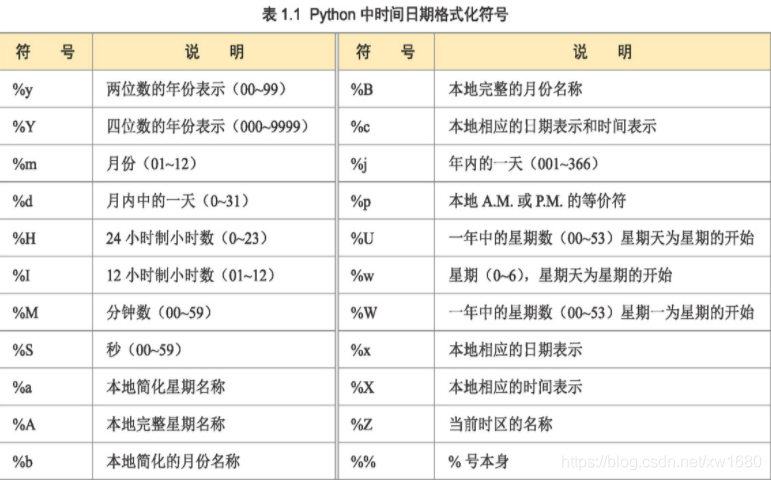1 2 3 4 5 6 7 8 9 10 11 12 13 14 15 16 17 18 19 20 21 22 23 24 25 26 27 28 29 30 31 32 33 34 35 36 37 38 39 40 41 42 43 44 45 46 47 48 49 50 `import` `datetime` `now ``=` `datetime.datetime.now()` `print``(``format``(now, ``'%Y-%m-%d %H:%M:%S %A'``)) ``# 当前时间格式化为年-月-日+完整英文星期` `print``(``format``(now, ``'%Y-%m-%d %H:%M:%S %a'``)) ``# 当前时间格式化为年-月-日+简写英文星期` `# 中文年-月-日显示` `print``(``format``(now, ``'%Y'``), ``'年'``, ``format``(now, ``'%m'``), ``'月'``, ``format``(now, ``'%d'``), ``'日'``)` `# 中文时间显示` `print``(``format``(now, ``'%H'``), ``'年'``, ``format``(now, ``'%M'``), ``'分'``, ``format``(now, ``'%S'``), ``'秒'``)` `print``(``format``(now, ``'%Y-%m-%d %H:%M:%S %a'``)) ``# 当前时间格式化为年-月-日+简写英文星期` `print``(``format``(now, ``'%Y-%m-%d'``)) ``# 当前时间格式化为标准年-月-日` `print``(``format``(now, ``'%y-%m-%d'``)) ``# 当前时间格式化为短日期年-月-日` `print``(``format``(now, ``'%Y<%m>%d'``)) ``# 当前时间格式化为长日期年-月-日，间隔符为“<”和“>”` `print``(``format``(now, ``'%c'``)) ``# 本地对应的年-月-日星期表示` `print``(``format``(now, ``'%B'``)) ``# 本地完整的月份表示，输出：May` `print``(``'现在是今年第'``, ``format``(now, ``'%j'``), ``'天'``) ``# 今天是一年中第几天，输出：现在是今年第 017 天` `print``(``'本周是今年第'``, ``format``(now, ``'%U'``), ``'周'``) ``# 本周是一年中第几周，输出：本周是今年第 02 周` `print``(``format``(now, ``'%y%m%d'``)) ``# 无间隔符短日期格式年月日，输出：210117` `print``(``format``(now, ``'%Y-%m'``)) ``# 长日期格式年-月，输出：2021-01` `print``(``format``(now, ``'%m-%d'``)) ``# 月-日显示，输出：01-17` `print``(``format``(now, ``'%m'``)) ``# 月份单独显示，输出：01` `print``(``format``(now, ``'%d'``)) ``# 日期单独显示，输出：17` `print``(``format``(now, ``'%H%M%S'``)) ``# 无间隔符，输出：133536` `print``(``format``(now, ``'%H:%M:%S'``)) ``# 标准时-分-秒，输出：13:35:36` `print``(``format``(now, ``'%I:%M:%S %I'``)) ``# 12小时制时-分-秒，输出：01:35:36 01` `print``(``format``(now, ``'%H:%M'``)) ``# 时+分，输出：13:35` `print``(``format``(now, ``'%M%S'``)) ``# 分+秒，输出：3536` `print``(``format``(now, ``'%H'``)) ``# 只显示小时，输出：13` `print``(``format``(now, ``'%H:%M:%S %p'``)) ``# 日期显示按AM，PM显示，输出：13:35:36 PM` `print``(``format``(now, ``'%a'``)) ``# 英文星期简写，输出：Sun` `print``(``format``(now, ``'%A'``)) ``# 英文星期完整显示，输出：Sunday` `week ``=` `[``'星期日'``, ``'星期一'``, ``'星期二'``, ``'星期三'``, ``'星期四'``, ``'星期五'``, ``'星期六'``]` `print``(week[``int``(``format``(now, ``'%w'``))]) ``# 中文星期，输出：星期日` `dt ``=` `datetime.datetime(``2020``, ``5``, ``9``)` `dm ``=` `datetime.datetime(``2020``, ``5``, ``9``, ``12``, ``50``, ``20``)` `# 将输入的日期按年-月-日和时间格式化，因时间没有输入，按0时处理` `print``(``format``(dt, ``'%Y-%m-%d %H:%M:%S'``))` `print``(``format``(dt, ``'%Y-%m-%d'``)) ``# 将输入的日期按年-月-日格式化` `print``(``format``(dm, ``'%Y-%m-%d %H:%M:%S'``)) ``# 将输入的日期按年-月-日和时间格式化` `print``(``format``(dm, ``'%Y-%m-%d'``)) ``# 将输入的日期按年-月-日格式化` `wx ``=` `datetime.datetime.now()` `print``(``str``(wx), ``format``(``1``, ``'0>3'``)) ``# 年-月-日 +3位编号` `print``(``format``(now, ``'%Y-%m-%d'``), ``format``(``1``, ``'0>3'``)) ``# 年-月-日 +3位编号` `print``(``format``(now, ``'%Y%m%d'``), ``'NO'` `+` `format``(``1``, ``'0>3'``)) ``# 年-月-日 +NO+3位编号` `print``(``format``(now, ``'%d'``), ``'NO'` `+` `format``(``1``, ``'0>3'``)) ``# 日期 +NO+3位编号` `print``(``format``(now, ``'%H%M'``), ``'NO'` `+` `format``(``1``, ``'0>3'``)) ``# 时钟+分 +NO+3位编号`

# 四、使用 f-string 方法

f-string 是 Python3.6 新增的一种字符串格式方法，由于前面已经介绍了多种格式化方式，大同小异，此处用简单的案例对其用法进行演示。

【示例1】使用 f-string 方法在字符串中嵌入变量和表达式

 1 2 3 4 `name ``=` `"Python"` `# 字符串` `ver ``=` `3.6` `# 浮点数` `# 输出：Python-3.6、Python-3.7、Python-3.8000000000000003` `print``(f``"{name}-{ver}、{name}-{ver + 0.1}、{name}-{ver + 0.2}"``)`

【示例2】在示例 1 中，表达式计算浮点数时发生溢出，可以使用特殊格式化修饰符限定只显示 1 位小数。

 1 2 3 4 `name ``=` `"Python"` `# 字符串` `ver ``=` `3.6` `# 浮点数` `# 输出：Python-3.6、Python-3.7、Python-3.8` `print``(f``"{name}-{ver}、{name}-{ver + 0.1}、{name}-{ver + 0.2:.1f}"``)`

【示例3】把十六进制数字 10 分别转换为用十进制、十六进制、八进制和二进制表示。

 1 2 3 `n ``=` `0x10` `# 十六进制数字10` `# 输出：dec:16, hex:10, oct:16, bin:10000` `print``(f``"dec:{n:d}, hex:{n:x}, oct:{n:0}, bin:{n:b}"``)`

【示例4】如果要在多行中表示字符串，可以使用下面示例方式，在每一行子串前面都加上 f 修饰符。

 1 2 3 4 5 `name ``=` `"Python"` `# 字符串` `ver ``=` `3.6` `# 浮点数` `s ``=` `f``"{name}-"` `\` ` ``f``"{ver}"` `print``(s) ``# 输出：Python-3.6`